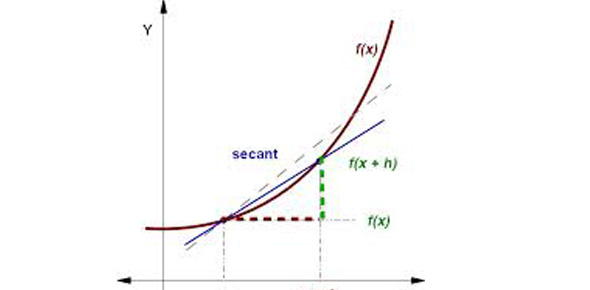# Limit Definition Of A Derivative

5 Questions | Total Attempts: 175SettingsThis quiz will test your knowledge of the limit definition of a derivative. You are to answer the questions to the best of your ability, and submit your answers no later than one week after the first lesson on the limit definition of a derivative.

• 1.
What is the formula for finding the derivative of a function over it's range?
• 2.
What is the formula for finding the derivative of a function at a point?
• 3.
Set up the limit to find the derivative of x^6+x^4+(x+3)^(1/2).  DO NOT SOLVE.
• 4.
What is the derivative of x^2?
• A.

X^2

• B.

2x

• C.

2x^2

• 5.
What is the derivative of x^4+3 at x=2?
• A.

32

• B.

4

• C.

12

• D.

3

Related TopicsBack to top